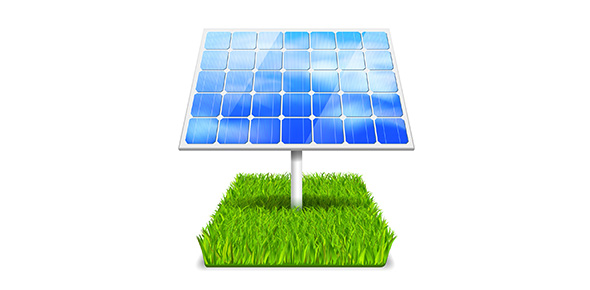# EMF, Potential Difference And Current

Approved & Edited by ProProfs Editorial Team
The editorial team at ProProfs Quizzes consists of a select group of subject experts, trivia writers, and quiz masters who have authored over 10,000 quizzes taken by more than 100 million users. This team includes our in-house seasoned quiz moderators and subject matter experts. Our editorial experts, spread across the world, are rigorously trained using our comprehensive guidelines to ensure that you receive the highest quality quizzes.
| By Patted03
P
Patted03
Community Contributor
Quizzes Created: 1 | Total Attempts: 403
Questions: 8 | Attempts: 404Settingsexplain and apply the concept of ‘electric current’ as the rate of flow of electric charge in an electric field—this will include applying the relationship: I=q/t
state that the direction of conventional current is that in which the flow of positive charge takes place, while the electron flow is in the opposite direction  Explain and apply the concepts of EMF and ‘potential difference’ as the loss of potential energy of electric charges as they flow through a circuit

• 1.

### A charge of 2 C flows through a wire over a period of 10 s. What is the current?

• A.

20 A

• B.

0.2 A

• C.

0.02 A

• D.

2.0 A

B. 0.2 A
Explanation
The current can be calculated by dividing the charge (2 C) by the time (10 s). Therefore, the current is 0.2 A.

Rate this question:

• 2.

### A charge of 2.5 C flows through a wire over a period of 5 s. What is the current?

• A.

50 A

• B.

0.5 A

• C.

10 A

• D.

0.1 A

B. 0.5 A
Explanation
The current can be calculated using the formula I = Q/t, where I is the current, Q is the charge, and t is the time. In this case, the charge is 2.5 C and the time is 5 s. Plugging these values into the formula, we get I = 2.5 C / 5 s = 0.5 A. Therefore, the correct answer is 0.5 A.

Rate this question:

• 3.

### A charge of 4 C flows through a wire over a period of 12 s. What is the current?

• A.

3 A

• B.

0.33 A

• C.

0.03 A

• D.

30 A

B. 0.33 A
Explanation
The current can be calculated using the formula I = Q/t, where I is the current, Q is the charge, and t is the time. In this case, the charge is 4 C and the time is 12 s. Substituting these values into the formula, we get I = 4 C / 12 s = 0.33 A. Therefore, the current is 0.33 A.

Rate this question:

• 4.

### A globe has a potential difference of 4 V. The current flowing through it is 2 A. How much energy is transferred to the globe in 30 s? Include units.

240J
240 J
Explanation
The energy transferred to the globe can be calculated using the formula: energy (E) = potential difference (V) x current (I) x time (t). Plugging in the given values, we get E = 4 V x 2 A x 30 s = 240 J. Therefore, the correct answer is 240J.

Rate this question:

• 5.

### A globe has a potential difference of 5 V. The current flowing through it is 1 A. How much energy is transferred to the globe in 30 s? Include units.

150J
150 J
Explanation
The energy transferred to the globe can be calculated using the formula: Energy = Potential difference × Current × Time. Given that the potential difference is 5 V, the current is 1 A, and the time is 30 s, we can substitute these values into the formula: Energy = 5 V × 1 A × 30 s = 150 J. Therefore, the energy transferred to the globe in 30 s is 150 Joules.

Rate this question:

• 6.

### A globe has a potential difference of 12 V. The current flowing through it is 4 A. How much energy is transferred to the globe in 30 s? Include units.

1440J
1440 J
Explanation
The energy transferred to the globe can be calculated using the formula: Energy = Voltage x Current x Time. Given that the potential difference is 12 V, the current is 4 A, and the time is 30 s, we can substitute these values into the formula. Energy = 12 V x 4 A x 30 s = 1440 J. Therefore, the energy transferred to the globe in 30 s is 1440 J.

Rate this question:

Related TopicsBack to top